# A Bayesian Model

A KDE is specified in terms of some function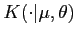where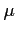is a location parameter and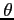, which may, more generally, be a vector, is a scale parameter. Examples of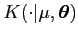include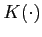a Normal pdf with locator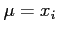and scale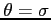. The estimate of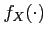based on the sample, at the point,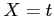is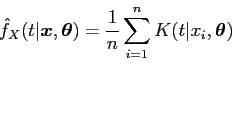(2)

where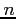is the number of data items. Note that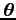may be considered to be a generalisation of bandwidth. Takingleads to the standard KDE of (1).

The form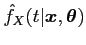exposes clearly the dependence of the estimator on both the sample to handand the parameter vectorand gives a density estimate that is a probability density if the kernelis a probability density. The estimate has the continuity of the kernel.

The need to choose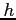, or more generally, is formulated as a problem of inference about. Sample dataand model (1) allow the building of a likelihood forand belief about the smoothness is expressed in a prior. Choice ofis provided by the posterior distribution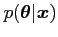.

By taking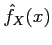to be a model for the data, a likelihood function can be constructed in the following way:

Define, as the sub-sample consisting of the first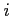elements of. Assuming no particular ordering to the sample data (so that it can, at least, be considered exchangeable with a random sample observed in the order implied by the subscripts), the likelihood may be written as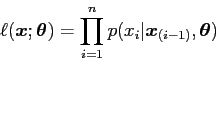(3)

where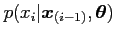is the probability density of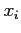, given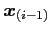and, given by the chosen model.

This is of the style commonly used for a time series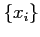, but is more generally true. If, for example, the density werewe would have the usual formulation for a random sample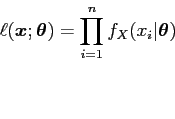(4)

If the KDE (2) is taken as a model for observationhaving seenthen (4) can be rewritten as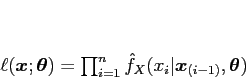(5)

It would be difficult to argue that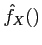is, in any sense, a true' model, but, in the absence of a parametric family for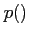, it is the best' available model.

Sinceis a parameter ofthere is generally some minimum number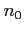(say) of values needed forto be defined and it seems reasonable to use a conditional likelihood of the form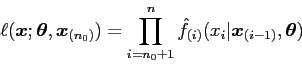(6)

The choice of priorwill, typically, reflect belief about the smoothness of the true density thatis intended to estimate. For example, for the basic KDE (1),is taken to be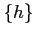. In this case a Normal prior forwith a high value ofimplies a belief in a rather smooth, near uniform density while a low value implies a non-smooth density. The variance parameter is chosen to reflect the strength of belief, a small value indicating a strongly held belief and a large value the opposite.

With small data sets the choice of prior has a large influence on the smoothness of the final estimate. It may be expected that with large data sets, for example those available from MCMC, the likelihood will dominate in the posterior and roughly non-informative priors will be a convenient initial choice.

Once the likelihood function and the prior are settled, Bayes theorem is applied to obtain the posterior density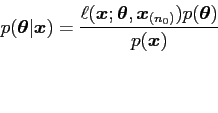(7)

in which(8)

where the integration is over the whole parameter space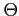.

The result in (7) is a posterior density for the parameter vector. The model used for the density is a KDE in which many densities are summed to model a single density. This is in no way anything like a realistic model since it depends on the sample size. It is not believed to be the true model, however the KDE family is very rich and adaptable, and it can be reasonably expected to provide an adequate model. It is, in any case, presumably the best available model, since otherwise some other density or approach would be used.

Situations in which the model is known, a priori, not to include the true' density have been considered by Berk (1966). In this situation the application of Bayes theorem leads, asymptotically, to the member nearest to the true' density, in terms of Kullback-Leibler directed distance (Kullback, 1997). Berk terms this the `asymptotic carrier'. There is then, some assurance that Bayes' methods will lead to an adequate, even optimal or near optimal, solution within the chosen modeling family -- in this case KDE.

The primary objective here is that of estimating the density. Within the family of KDEs, defined in terms of, it is easy to obtain estimates of the form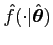, where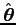is some convenient point estimate of, perhaps the posterior mode or the posterior mean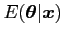. Such a density could be evaluated at some set of values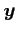, as, and this estimative density plotted. Hereis an estimate, not the true value, and the estimate makes no allowance for the shape (or uncertainty in the estimate) of the posterior distribution for.

A better approach is to integrate over the parameter space ofgiving the predictive density of the unobserved data. See, for example Aitchison and Dunsmore (1975):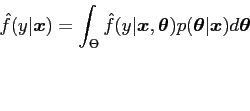(9)

Each point requires the evaluation of an integral like (9) for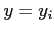- unfortunately, this becomes computationally expensive. Given data with range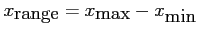, a set of points is chosenthat give sufficient information to cover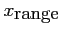. The corresponding values of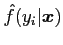are plotted.

In (8) and (9) the integration is over the whole parameter space, this is typically of low dimension but in many cases it is not possible to obtain the result analytically. In such cases numerical quadrature 1 see Naylor and Smith (1982) can be applied.

danny 2009-07-23